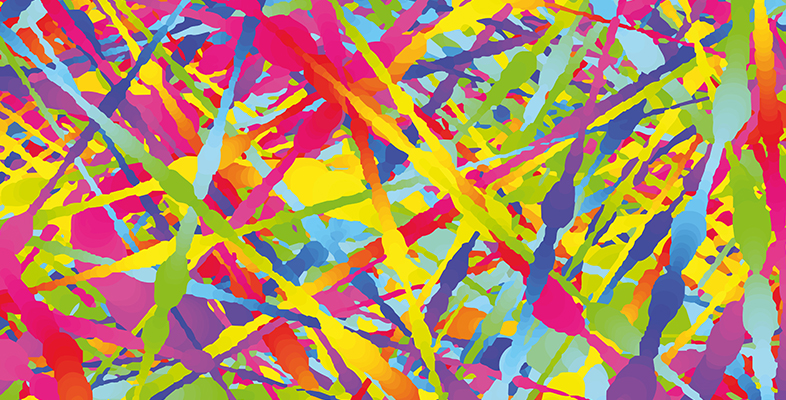Science, Maths & Technology

### Become an OU studentExploring communications technology

Start this free course now. Just create an account and sign in. Enrol and complete the course for a free statement of participation or digital badge if available.

In ASK, only the amplitude of the carrier signal is modified in modulation. The simplest version is on–off keying (OOK). In OOK, either bursts of a carrier wave are transmitted or nothing is transmitted depending whether the input message signal is 1 or 0. Other versions of ASK use differing (non-zero) amplitudes to represent 1 and 0.

Figure 1.2(a) shows a digital message signal using two voltage levels. One level represents 1 and the other represents 0. The unmodulated carrier is illustrated in Figure 1.2(b). Figure 1.2(c) and (d) are the modulated waveforms using two versions of ASK. Figure 1.2(c) uses OOK, and 2(d) uses binary ASK, or BASK.Figure 1.2 ASK: (a) data; (b) unmodulated carrier; (c) on–off keying (OOK); (d) binary amplitude-shift keying (BASK)

In OOK and BASK, the modulated carrier can take one of two different states: one state representing a 0, the other a 1. These different carrier states are what are known as symbols. If there are more than two possible carrier states – that is, more than two symbols available – then it is possible for each symbol to represent more than one bit.

Figure 1.3 shows ASK with four possible amplitude levels, or four symbols. With four symbols available, each symbol can be uniquely represented with a two-bit binary number. This is because there are just four possible two-bit binary numbers: 11, 10, 01 and 00.Figure 1.3 ASK with four amplitude levels

If there were eight symbols, each could represent three data bits. The relationship between the number of available symbols, M, and the number of bits that can be represented by a symbol, n, is:

M = 2n

The term baud refers to the number of symbols per second, where one baud is one symbol per second.

Data rate (or bit rate) and baud are closely related.

## Activity 1.4 Self assessment

• a.If a communications system uses 16 symbols, how many bits does each symbol represent?
• b.If the same system has a symbol rate of 10 000 baud, what is the data rate?